Refer to our Texas Go Math Grade 6 Answer Key Pdf to score good marks in the exams. Test yourself by practicing the problems from Texas Go Math Grade 6 Unit 3 Study Guide Review Answer Key.

Module 7 Representing Ratios and Rates

Write three equivalent ratios for each ratio.

Question 1.
$$\frac{18}{6}$$
Solution to this example is given below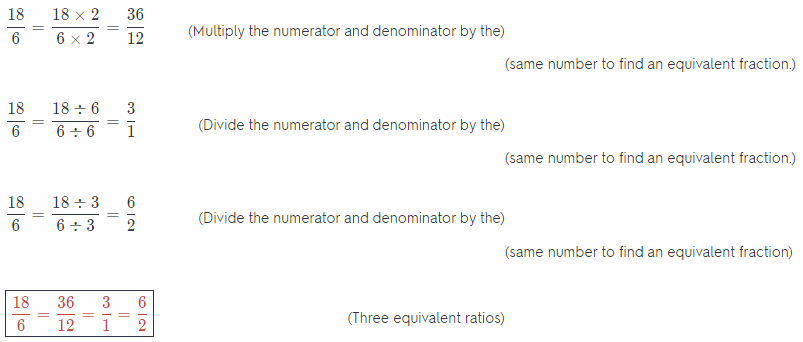Question 2.
$$\frac{5}{45}$$
Solution to this example is given below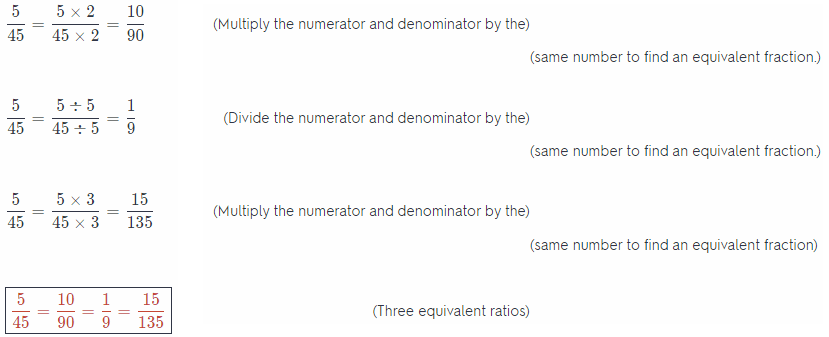Question 3.
$$\frac{3}{5}$$
Given ratio: $$\frac{3}{5}$$

Evaluate an equivalent ratio of the given ratio by multiplying both the numerator and the denominator of the given ratio with the same number, therefore:
$$\frac{3}{5}=\frac{3 \times 2}{5 \times 2}=\frac{6}{10}$$

And:
$$\frac{3}{5}=\frac{3 \times 3}{5 \times 3}=\frac{9}{15}$$

And:
$$\frac{3}{5}=\frac{3 \times 4}{5 \times 4}=\frac{12}{20}$$

$$\frac{3}{5}=\frac{6}{10}=\frac{9}{15}=\frac{12}{20}$$

Question 4.
To make a dark orange color, Ron mixes 3 ounces of red paint with 2 ounces of yellow paint. Write the ratio of red paint to yellow paint three ways. (lesson 7.1)
The ratio of red paint to yellow paint is 3 : 2 = $$\frac{3}{2}$$
Expand that fraction (multiply its numerator and denominator with the same number) to get fractions equivalent to it, i.e. to get ratios equivalent to 3 : 2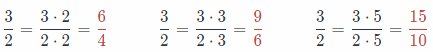So, the corresponding equivalent ratios of red paint to yellow paint are:
6 : 4, 9 : 6, and 15: 10

Question 5.
A box of a dozen fruit tarts costs $15.00. What is the cost of one fruit tart? (lesson 7.2) Answer: 1 dozen contains 12 units. Here this implies that 12 fruit tarts cost$ 15 so the cost of 1 fruit tart is equal to $$\frac{15}{12}$$ = $1.25 The cost of 1 fruit tart is$ 1.25

Compare the ratios.

Question 6.
$$\frac{2}{5}$$ ____________ $$\frac{3}{4}$$
It can be seen that the denominators of the given fractions are not equal so they can not be directly compared.
Therefore, evaluate the decimal equivalent of the given fractions to compare them, therefore:
$$\frac{2}{5}$$ = 0.4
And:
$$\frac{3}{4}$$ = 0.75
Here 0.4 < 0.75 so $$\frac{2}{5}$$ < $$\frac{3}{4}$$

Question 7.
$$\frac{9}{2}$$ ____________ $$\frac{10}{7}$$
Expand the given fractions so they have common denominators (the best candidate is always the lCM of those denominators):Now we easily see that
$$\frac{9}{2}=\frac{63}{14}$$ > $$\frac{20}{14}=\frac{10}{7}$$

Question 8.
$$\frac{2}{11}$$ ____________ $$\frac{3}{12}$$
It can be seen that the denominators of the given fractions are not equal so they can not be directly compared.
Therefore, evaluate the decimal equivalent of the given fractions to compare them, therefore:
$$\frac{2}{11}$$ = $$0 . \overline{1} \overline{8}$$
And:
$$\frac{3}{12}$$ = 0.25
Here $$0 . \overline{1} \overline{8}$$ < 0.25 so $$\frac{2}{11}$$ < $$\frac{3}{12}$$

Question 9.
$$\frac{6}{7}$$ ____________ $$\frac{8}{9}$$
Expand the given fractions so they have common denominators (the best candidate is always the lCM of those denominators):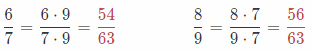Now we easily see that
$$\frac{6}{7}=\frac{54}{63}$$ < $$\frac{56}{63}=\frac{8}{9}$$
$$\frac{6}{7}$$ < $$\frac{8}{9}$$

Module 8 Applying Ratios and Rates

Question 1.
Thaddeus already has $5 saved. He wants to save more to buy a book. Complete the table, and graph the ordered pairs on the coordinate graph. (lesson 8.1, 8.2)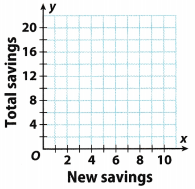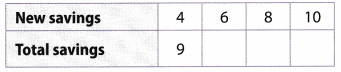Answer:  New savings Total savings 4 9 6 11 8 13 10 15 Total savings = New Savings + 5 list the ordered pairs from the table: (4, 9), (6, 11), (8, 13), (10, 15) and graph them on a coordinate plane.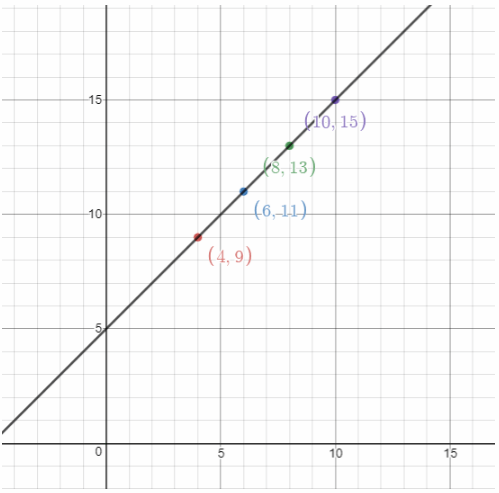Question 2. There are 2 hydrogen atoms and 1 oxygen atom in a water molecule. Complete the table, and list the equivalent ratios shown on the table. (lesson 8.1, 8.2)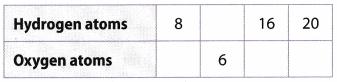Answer:  New savings Total savings 8 4 12 6 16 8 20 10 Hydrogen atoms = Oxygen atoms ∙ 2 Equivalent ratios: $$\frac{8}{4}=\frac{12}{6}=\frac{16}{8}=\frac{20}{10}$$ Question 3. Sam can solve 30 multiplication problems in 2 minutes. How many can he solve in 20 minutes? (lesson 8.3) Answer: To find the unit rate, divide the numerator and denominator by 2: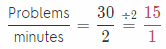He can solve 15 problems in 1 minute. Therefore, in 20 minutes he can solve 20 ∙ 15 = 300 problems Question 4. A male Chihuahua weighs 5 pounds. How many ounces does he weigh? Answer: 1 pound = 16 ounces ⇒ 5 ∙ 16 = 80 ounces Module 9 Percents Write each fraction as a decimal and a percent. (lessons 9.1, 9.2) Question 1. $$\frac{3}{4}$$ Answer: Write an equivalent fraction with a denominator of 100 $$\frac{3}{4}=\frac{3 \times 25}{4 \times 25}=\frac{75}{100}$$ (Multiply both the numerator and denominator by 25) Write the decimal equivalent $$\frac{75}{100}$$ = 0.75 Write the percent equivalent. $$\frac{75}{100}$$ = 0.75 = 75% (Move the decimal point 2 places to the right) Final Solution ⇒ $$\frac{3}{4}$$ = 0.75 = 75% Question 2. $$\frac{7}{20}$$ Answer: Write an equivalent fraction with a denominator of 100 $$\frac{7}{20}=\frac{7 \times 5}{20 \times 5}=\frac{35}{100}$$ (Multiply both the numerator and denominator by 25) Write the decimal equivalent $$\frac{35}{100}$$ = 0.35 Write the percent equivalent. $$\frac{35}{100}$$ = 0.35 = 35% (Move the decimal point 2 places to the right) Final solution ⇒ $$\frac{7}{20}$$ = 0.35 = 35% Question 3. $$\frac{8}{5}$$ Answer: Write an equivalent fraction with a denominator of 100 $$\frac{8}{5}=\frac{8 \times 20}{5 \times 20}=\frac{160}{100}$$ (Multiply both the numerator and denominator by 25) Write the decimal equivalent $$\frac{160}{100}$$ = 1.60 Write the percent equivalent. $$\frac{160}{100}$$ = 1.60 = 160% (Move the decimal point 2 places to the right) Final solution ⇒ $$\frac{8}{5}$$ = 1.6 = 160% Complete each statement. (lessons 9.1, 9.2) Question 4. 25% of 200 is ______________ . Answer: Explanation A: Multiply by a fraction to find 25 % of 200 Write the percent as a fraction. Multiply $$\frac{25}{100}$$ of 200 = $$\frac{25}{100}$$ × 200 = $$\frac{5000}{100}$$ = 50 Final Solution = 50 25% of 200 is 50 Explanation B Data: Portion = x Total = 200 Percent = 25 Write equation of percentage: Percent = $$\frac{\text { Portion }}{\text { Total }}$$ × 100% Substitute values: 25% = $$\frac{x}{200}$$ × 100% Solve for x: x = $$\frac{200 \times 25 \%}{100 \%}$$ Evaluate: x = 50 25% of 200 is 50 Question 5. 16 is ___________ of 20. Answer: Data: Portion = 16 Total = 20 Percent = x Write equation of percentage: Percent = $$\frac{\text { Portion }}{\text { Total }}$$ × 100% Substitute values: x = $$\frac{16}{20}$$ × 100% Evaluate: x = 80% Explanation B: Multiply by a fraction to find 16 is x of 20 Write the percent as a fraction. Multiply $$\frac{16}{20}$$ of 100 = $$\frac{16}{20}$$ × 100% = $$\frac{1600}{20}$$ = 80% Final Solution = 80% 16 is 80% of 20 Question 6. 21 is 70% of ______________ . Answer: Multiply by a fraction to find 21 is 70% of x Write the percent as a fraction. Multiply $$\frac{21}{70}$$ of 100 = $$\frac{21}{70}$$ × 100% = $$\frac{2100}{70}$$ = 30 Final Solution = 30 21 is 70% of 30 Explanation B Data: Portion = 21 Total = x Percent = 70 Write equation of percentage: Percent = $$\frac{\text { Portion }}{\text { Total }}$$ × 100% Substitute values: 70% = $$\frac{21}{x}$$ × 100% Solve for x: x = $$\frac{21 \times 100 \%}{70 \%}$$ Evaluate: x = 30 21 is 70% of 30 Question 7. 42 of the 150 employees at Carlo’s Car Repair wear contact lenses. What percent of the employees wear contact lenses? (lesson 9.3) Answer: Multiply by a fraction to find 42 is x of 50 Write the percent as a fraction. Multiply x = $$\frac{42}{150}$$ of 100 = $$\frac{42}{150}$$ × 100% x = $$\frac{4200}{150}$$ x = 28% Final Solution = 28% 42 is 28% of 150 Explanation B Data: Portion = 42 Total = 150 Percent = x Write equation of percentage: Percent = $$\frac{\text { Portion }}{\text { Total }}$$ × 100% Substitute values: x = $$\frac{42}{150}$$ × 100% Evaluate: x = 28% 28% of the employees at Carlo’s Car Repair wear Contact lenses. Question 8. last week at Best Bargain, 75% of the computers sold were laptops. If 340 computers were sold last week, how many were laptops? (lesson 9.3) Answer: Multiply by a fraction to find 75% of 340 Write the percent as a fraction. Multiply x = $$\frac{75}{100}$$ of 340 = $$\frac{75}{100}$$ × 340 x = $$\frac{25500}{100}$$ x = 255 Final Solution = 255 75% of 340 is 255 255 laptops were sold last week. Explanation B Data: Portion = x Total = 340 Percent = 75 Write equation of percentage: Percent = $$\frac{\text { Portion }}{\text { Total }}$$ × 100% Substitute values: 75% = $$\frac{x}{340}$$ × 100% Solve for x: x = $$\frac{340 \times 75 \%}{100 \%}$$ Evaluate: x = 255 21 is 70% of 30 255 laptops were sold. Texas Go Math Grade 6 Unit 3 Performance Task Answer Key Question 1. CAREERS IN MATH Residential Builder Kaylee, a residential builder, is working on a paint budget for a custom-designed home she is building. A gallon of paint costs$38.50, and its label says it covers about 350 square feet.

a. Explain how to calculate the cost of paint per square foot. Find this value. Show your work.
To find the unit rate, divide the numerator and denominator by 350The cost per square foot is
$0.11 b. Kaylee measured the room she wants to paint and calculated a total area of 825 square feet. If the paint is only available in one-gallon cans, how many cans of paint should she buy? Justify your answer. Answer: $$\frac{825}{350}$$ = 2.35 ⇒ 3 cans She should buy 3 cans (because she can not buy 2.35 cans, and if she buy 2 cans, she would not have enough for the whole room). Question 2. Davette wants to buy flannel sheets. She reads that a weight of at least 190 grams per square meter is considered high quality. a. Davette finds a sheet that has a weight of 920 grams for 5 square meters. Does this sheet satisfy the requirement for high-quality sheets? If not, what should the weight be for 5 square meters? Explain. Answer: To find the unit rate, divide the numerator and denominator by 5:Since 184 < 190, this sheet does not satisfy the requirement for high-quality sheets. The weight should be 5 ∙ 190 = 950 b. Davette finds 3 more options for flannel sheets: Option 1:1,100 g of flannel in 6 square meters,$45
Option 2: 1,260 g of flannel in 6.6 square meters, $42 Option 3: 1,300 g of flannel in 6.5 square meters,$52
She would like to buy the sheet that meets her requirements for high quality and has the lowest price per square meter. Which option should she buy? Justify your answer.
Option 1:
To find the unit rate, divide the numerator and denominator by 6:Since 183.33 < 190, this sheet does not satisfy the requirement for high-quality sheets.

Option 2:
To find the unit rate, divide the numerator and denominator by 6.6: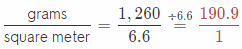Since 190.9 > 190, this sheet does satisfy the requirement for high-quality sheets.
To find the unit rate, divide the numerator and denominator by 6.6: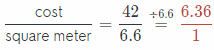The price per square meter is
= $6.361 Option 3: To find the unit rate, divide the numerator and denominator by 6.5: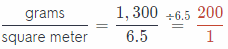Since 200 > 190, this sheet does satisfy the requirement for high-quality sheets.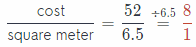The price per square meter is$8

Texas Go Math Grade 6 Unit 1 Texas Test Prep Answer Key

Selected Response

Question 1.
The deepest part of a swimming pool is 12 feet deep. The shallowest part of the pool is 3 feet deep. What is the ratio of the depth of the deepest part of the pool to the depth of the shallowest part of the pool?
(A) 4:1
(B) 12:15
(C) 1:4
(D) 15:12
(A) 4 : 1

Explanation:
The deepest part of a swimming pool is 12 feet deep The shallowest part of the pool is 3 feet deep. Therefore, the ratio of the depth of the deepest part of the pool to the depth of the shallowest part of the pool is 12 : 3. This in fraction form is equal to $$\frac{12}{3}=\frac{4}{1}$$ = 4 : 1.

Question 2.
How many centimeters are in 15 meters?
(A) 0.15 centimeters
(B) 1.5 centimeters
(C) 150 centimeters
(D) 1,500 centimeters
(D) 1,500 centimeters

Explanation:
There are 100 centimeters in 1 meter. Therefore in 15 meters there are 15 × 100 = 1500 centimeters.

Question 3.
Barbara can walk 3,200 meters in 24 minutes. How far can she walk in 3 minutes?
(A) 320 meters
(B) 400 meters
(C) 640 meters
(D) 720 meters
(B) 400 meters

Explanation:
Barbara can walk 3,200 meters in 24 minutes This implies that her rate is $$\frac{3200}{24}$$ = 133.$$\overline{3}$$ meters per minute.
Therefore, she can walk 133.$$\overline{3}$$ × 3 = 400 meters in 3 minutes.

Question 4.
The table below shows the number of windows and panes of glass in the windows.Which represents the number of panes?
(A) windows × 5
(B) windows × 6
(C) windows + 10
(D) windows + 15
(B) windows × 6

Explanation:
It can be seen that the number of panes is always 6 times that of the windows, therefore Option B.

Question 5.
The graph below represents Donovan’s speed while riding his bike.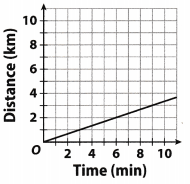Which would be an ordered pair on the line?
(A) (1,3)
(B) (2, 2)
(C) (6,4)
(D) (9, 3)
(D) (9, 3)

Explanation:
It can be seen that only point (9, 3) lies on the line.

Hot Tip! Read the graph or diagram as closely as you read the actual test question. These visual aids contain important information.

Question 6.
Which percent does this shaded grid represent?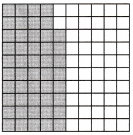(A) 42%
(B) 48%
(C) 52%
(D) 58%
(B) 48%

Explaination:
Count the number of shaded boxes. It can be seen that 48 out of 100 are shaded, this means that 48% of them are shaded.

Question 7.
Ivan saves 20% of his monthly paycheck for music equipment. He earned $335 last month. How much money did Ivan save for music equipment? (A)$65
(B) $67 (C)$70
(D) $75 Answer: (B)$67

Explanation:
Multiply by a fraction to find 20% of 335
Write the percent as a fraction.

Multiply
x = $$\frac{20}{100}$$ of 335 = $$\frac{20}{100}$$ × 355
x = $$\frac{6700}{100}$$
x = 67

20% of 335 is 67
Ivan saves 67 dollars

Question 8.
How many 0.6-liter glasses can you fill up with a 4.5-liter pitcher?
(A) 1.33 glasses
(B) 3.9 glasses
(C) 7.3 glasses
(D) 7.5 glasses
(D) 7.5 glasses

Explanation:
The divisor has one decimal place, so multiply both the dividend and the divisor by 10 so that the divisor is a whole number
0.6 × 10 = 6
4.5 × 10 = 45

Divide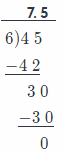Question 9.
Which shows the integers in order from greatest to least?
(A) 22, 8, 7, 2, – 11
(B) 2, 7, 8, – 11, 22
(C) – 11, 2, 7, 8, 22
(D) 22, – 11, 8, 7, 2
(A) 22, 8, 7, 2, – 11

Explanation:
We can easily see that A) is correct because:
22 > 8 > 7 > 2 > – 11

Let’s check the other options:
B) is incorrect because, for example: 2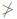7
C) is incorrect because, for example: – 112
D) is incorrect because, for example: – 118

Note: There was not really any need to check the other options once we noticed that the same 5 numbers appeared in all options – one set of numbers can be ordered from greatest to least in I was only

Gridded Response

Question 10.
Melinda bought 6 bowls for $13.20. What was the unit rate, in dollars?Answer: To find the unit rate, divide the numerator and denominator by 6: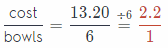The cost per bowl is$2.2

Question 11.
A recipe calls for 6 cups of water and 4 cups of flour. If the recipe is increased, how many cups of water should be used with 6 cups of flour?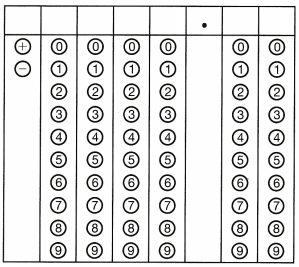$$\frac{\text { water }}{\text { flour }}=\frac{6}{4}=\frac{x}{6}$$
6 is a common denominator:
$$\frac{6}{4} \cdot \frac{1.5}{1.5}=\frac{x}{6}$$
$$\frac{9}{6}=\frac{x}{6}$$
⇒ x = 9

9 cups of water.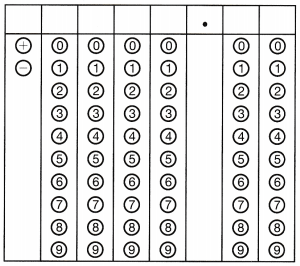⇒ $$\frac{4}{25}$$ = 016 = 16%